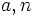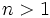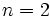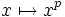# Perfect (mathematics)

## Contents

### In group theory

Perfect group: A group that equals its own commutator subgroup (i.e. derived subgroup).

Main subject wiki entry: Groupprops:Perfect group

Also located at: Wikipedia:Perfect group, Mathworld:PerfectGroup, Planetmath:PerfectGroup

### In topology

Perfect space: A topological space where every point is closed, and is an intersection of countably many open subsets containing it.

Main subject wiki entry: Topospaces:Perfect space

Perfectly normal space: A normal space where every closed subset is an intersection of countably many open subsets containing it.

Primary subject wiki entry: Topospaces:Perfectly normal space

Perfect set: A set in a metric space that has no isolated points.

### In number theory

Perfect power: A natural number expressible as, whereare natural numbers andFor, termed a perfect square. For, termed a perfect cube.

No relevant subject wiki entry.

Also located at: Wikipedia:Perfect power, Mathworld:PerfectPower

Perfect number: A natural number that equals the sum of all its proper (positive) divisors.

Primary subject wiki entry: Number:Perfect number

Also located at: Wikipedia:Perfect number, Mathworld:PerfectNumber, Planetmath:PerfectNumber

### In field theory

Perfect field: A field that either has characteristic zero, or has characteristicand for which the mapis a surjective map. Equivalently, it is a field such that every algebraic extension field for it is separable.

Primary subject wiki entry: Galois:Perfect field

Also located at: Mathworld:PerfectField, Planetmath:PerfectField

### In graph theory

Perfect graph: A graph with the property that for every induced subgraph, the chromatic number equals the clique number.

Term variations: Strongly perfect graph

No relevant subject wiki entry.

Also located at: Wikipedia:Perfect graph, Mathworld:PerfectGraph

Perfect matching: A matching in a bipartite graph such that every element on one side gets matched to exactly one element on the other side.

No relevant subject wiki entry.